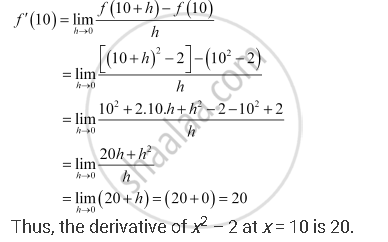CBSE (Arts) Class 11CBSE
Share

# Find the Derivative of X2 – 2 at X = 10. - CBSE (Arts) Class 11 - Mathematics

ConceptDerivative Algebra of Derivative of Functions

#### Question

Find the derivative of x2 – 2 at x = 10.

#### Solution

Let f(x) = x2 – 2. Accordingly,Is there an error in this question or solution?

#### APPEARS IN

NCERT Solution for Mathematics Textbook for Class 11 (2018 to Current)
Chapter 13: Limits and Derivatives
Q: 1 | Page no. 312

#### Video TutorialsVIEW ALL 

Solution Find the Derivative of X2 – 2 at X = 10. Concept: Derivative - Algebra of Derivative of Functions.
S